【欢迎转载，请注明作者：房燕良，原文出处：游戏程序员的自我修养

## PBR 从离线渲染进入游戏引擎

PBR 对于现代 3D 引擎十分重要，它使得实时渲染突破了被常年诟病的“塑料感”，我们有了金属、皮革、瓷器！以从业者的角度看，更重要的是：它使我们可以把材质与光照解耦。也就是说一个 PBR 材质，在不同的光照环境下都应该得到正确的渲染结果，这让 3D 美术工作者可以更方便的调节对象的材质，并使得材质更具备通用性。

## 理解 PBR 的原理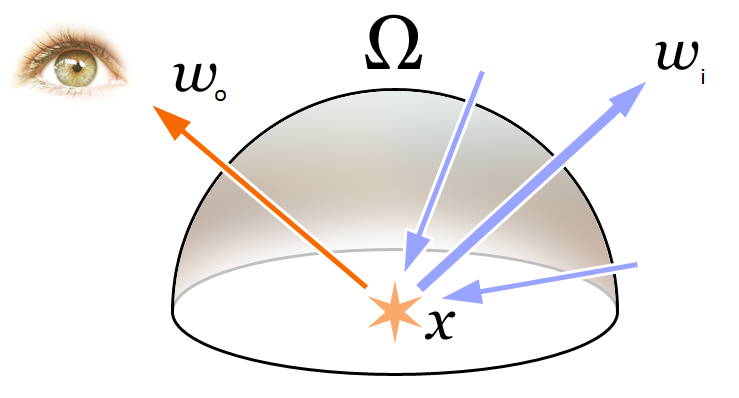James T. Kajiya 在 SIGGRAPH 1986 中发表的论文《The Rendering Equation》中提出了这个概念。文章首先高屋建瓴的从辐射度量学的角度，用一个方程描述光在场景中的转播，并给出了下面这个方程，并且提出了使用蒙特卡洛方法求它的数值解的思路，并命名为 Path Tracing！ 另外，文章标题中的冠词用的是 “The” ，而不是 “A”，实际是确认也是如此，后面的发展方向基本也是以这个方程为出发点了。

$L_o(p, v) = \int\limits_H L_i(p,l) f(l, v) n \cdot l dl$

• $L$：辐射率（Radiance），这是一个物理概念，下面我用一小节专门解释
• $p$：物体表面上的一个点
• $v$：观察方向
• $l$：入射光方向
• $f$：BRDF，非常重要的概念，后面单独一小节解释
• $\int\limits_H … dl$：在半球域（Hemisphere）内，对所有入射光线进行积分
• $n$：这个最简单了，就是$p$点所在平面的法向量啦

### BRDF

BRDF 是 Bidirectional Reflective Distribution Function 的缩写，中文译为双向反射分布函数。这个概念由 Fred E. Nicodemus 于1965年提出。

### 立体角（Solid Angle）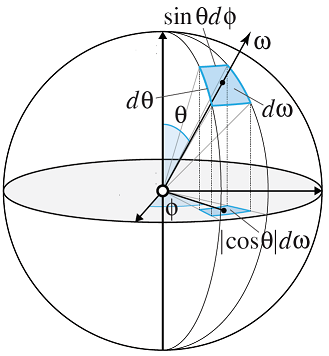$d\omega=\sin\theta\;d\theta\;d\phi\;$

## Cook-Torrance 反射模型

$R_{bd} = k_d R_d + k_s R_s$

$R_d = \frac{c_{diff}}{\pi}$

$R_s = \frac{D(h)F(l,h)G(l,v,h)}{4 (n \cdot l)(n \cdot v)}$

• D：微平面在平面上的分布函数
• G：计算微平面由于互相遮挡而产生的衰减
• F：菲涅尔项

### 微平面模型（The Microfacet Model）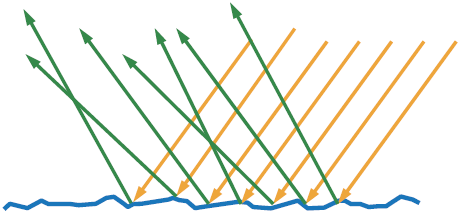### 镜面反射$R_s$中的$D$、$F$、$G$

#### D：微平面分布函数（Normal distribution function）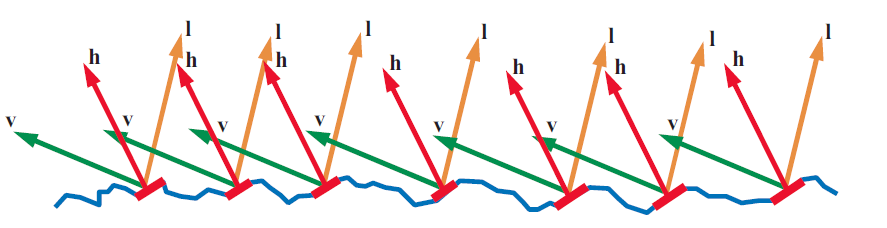$D(h) = \frac{\alpha^2}{\pi((n \cdot h)^2 (\alpha^2 - 1) + 1)^2}$

• $n$ 为表面的宏观法向量
• $h$ 入射光和观察方向的中间向量
• $\alpha$ 为表面的粗糙度参数

#### G：几何遮挡因子（Geometric Occlusion Factor）

$k = \frac{(\alpha + 1)^2}{8}$ $G_1(v) = \frac{n \cdot v}{(n \cdot v)(1 - k) + k }$ $G(l, v, h) = G_1(1) G_1(v)$

#### F：菲涅尔项（Fresnel Factor）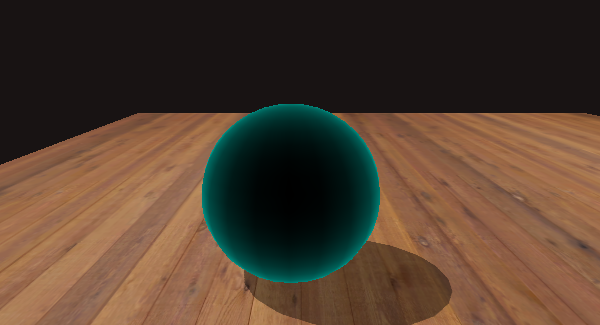$F(v, h) = F_0 +(1-F_0)2^{(-5.55473(v \cdot h)-6.98316)(v \cdot h)}$